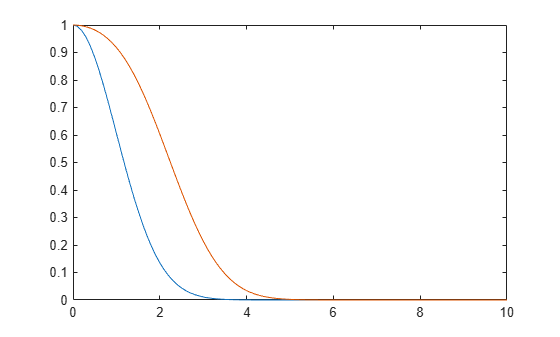# marcumq

Generalized Marcum Q-function

## Syntax

``Q = marcumq(a,b)``
``Q = marcumq(a,b,m)``

## Description

example

````Q = marcumq(a,b)` computes the Marcum Q-function of order 1 for noncentrality parameter `a` and argument `b`. Use this syntax to calculate the special case of the generalized Marcum Q-function of order m with m = 1. For the definition of the generalized Marcum Q-function, see Generalized Marcum Q-function.```
````Q = marcumq(a,b,m)` computes the generalized Marcum Q-function of order `m`.```

## Examples

collapse all

Specify a range of values over which to generate data for the Marcum $Q$-function.

`b = (0:0.1:10)';`

Generate two output vectors comprising values of the Marcum $Q$-function of order one with noncentrality parameter values of `0` and `2`.

```Q1 = marcumq(0,b); Q2 = marcumq(2,b);```

Plot the resultant Marcum $Q$-functions.

`plot(b,[Q1 Q2])`## Input Arguments

collapse all

Noncentrality parameter, specified as an array of nonnegative numbers.

Note

If you specify any input as an array, you must specify all other inputs as arrays of the same size or as scalars. If you specify any of the inputs as a scalar, the function expands that input to match the size of the other inputs.

Data Types: `double`

Argument of the Marcum Q-function, specified as an array of nonnegative numbers.

Data Types: `double`

Order of the generalized Marcum Q-function, specified as an array of positive integers.

Data Types: `double`

## Output Arguments

collapse all

Value of the Marcum Q-function, returned as an array of numbers in the range [0, 1]. The size of this output matches the size of the input arguments.

Data Types: `double`

collapse all

### Generalized Marcum Q-function

The generalized Marcum Q-function of order m is defined by

`${Q}_{m}\left(a,b\right)=\frac{1}{{a}^{m-1}}{\int }_{b}^{\infty }{x}^{m}\mathrm{exp}\left(-\frac{{x}^{2}+{a}^{2}}{2}\right){I}_{m-1}\left(ax\right)dx,$`

where Im – 1 is the modified Bessel function of the first kind of order m – 1.

## Algorithms

To compute the value of the generalized Marcum Q-function for the specified inputs, this function uses a calculation based on a method illustrated by Shnidman in . This paper describes two error criteria: a relative error criterion and an absolute error criterion. This function uses the absolute error criterion.

 Cantrell, P., and A. Ojha. “Comparison of Generalized Q-function Algorithms (Corresp.).” IEEE® Transactions on Information Theory 33, no. 4 (July 1987): 591–96. https://doi.org/10.1109/TIT.1987.1057323.

 Marcum, J. “A Statistical Theory of Target Detection by Pulsed Radar.” IEEE Transactions on Information Theory 6, no. 2 (April 1960): 59–267. https://doi.org/10.1109/TIT.1960.1057560.

 Shnidman, D.A. “The Calculation of the Probability of Detection and the Generalized Marcum Q-function,” IEEE Transactions on Information Theory 35, no. 2 (March 1989): 389–400. https://doi.org/10.1109/18.32133.